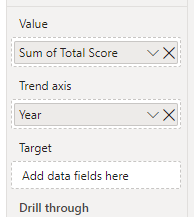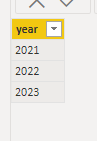cancel
Showing results for
Did you mean:Helper I

## DAX in KPI

Hi,

I would like to have a measure to present this idea but I don't really know how to do it with DAX, please see if it is feasible or not, thank you.

if year == 2021:

target_score = 2021's score

else:

target_score = year-1's score

For example, if the slicer selects 2021, the comparison could be (+0.0) if the slicer selects 2022. the KPI visual will compare the score of 2022 vs 2021 (2022-1). I would like to put the measure to the Target field in the KPI visual.1 ACCEPTED SOLUTIONSuper User

@gdxy , Create a separate year/date table and have a measure like

//Only year vs Year, not a level below

This Year = CALCULATE(sum('Table'[Qty]),filter(ALL('Date'),'Date'[Year]=max('Date'[Year])))
Last Year = CALCULATE(sum('Table'[Qty]),filter(ALL('Date'),'Date'[Year]=max('Date'[Year])-1))
diff = [This Year]-[Last Year ]
diff % = divide([This Year]-[Last Year ],[Last Year ])

Time Intelligence, DATESMTD, DATESQTD, DATESYTD, Week On Week, Week Till Date, Custom Period on Period,
Custom Period till date: https://youtu.be/aU2aKbnHuWs&t=145s

Power BI — Year on Year with or Without Time Intelligence
https://medium.com/@amitchandak.1978/power-bi-ytd-questions-time-intelligence-1-5-e3174b39f38a

4 REPLIES 4Super User

@gdxy , Create a separate year/date table and have a measure like

//Only year vs Year, not a level below

This Year = CALCULATE(sum('Table'[Qty]),filter(ALL('Date'),'Date'[Year]=max('Date'[Year])))
Last Year = CALCULATE(sum('Table'[Qty]),filter(ALL('Date'),'Date'[Year]=max('Date'[Year])-1))
diff = [This Year]-[Last Year ]
diff % = divide([This Year]-[Last Year ],[Last Year ])

Time Intelligence, DATESMTD, DATESQTD, DATESYTD, Week On Week, Week Till Date, Custom Period on Period,
Custom Period till date: https://youtu.be/aU2aKbnHuWs&t=145s

Power BI — Year on Year with or Without Time Intelligence
https://medium.com/@amitchandak.1978/power-bi-ytd-questions-time-intelligence-1-5-e3174b39f38aHelper I

Not sure why, but when I use
Last Year = CALCULATE(sum('university average (3)'[Value]),filter(ALL('year'),'year'[year]=MAX('year'[year])-1))
nothing is shown

Here are my tables:Helper I

I assume when I put the measure to the 'Target' field, if the year is 2021, the value will be the score in 2021, when the year is 2022 or above, the value will be the score of last year.Helper I

Sorry, I figured out the reason, the data type is text in one of my data fields.